# Bootstrap method

(diff) ← Older revision | Latest revision (diff) | Newer revision → (diff)

A computer-intensive "resampling" method, introduced in statistics by B. Efron in 1979 [a3] for estimating the variability of statistical quantities and for setting confidence regions (cf. also Sample; Confidence set). The name "bootstrap" refers to the analogy with pulling oneself up by one's own bootstraps. Efron's bootstrap is to resample the data. Given observations, artificial bootstrap samples are drawn with replacement from, putting equal probability mass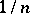at each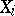. For example, with sample size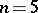and distinct observationsone might obtain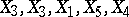as bootstrap (re)sample. In fact, there are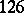distinct bootstrap samples in this case.

A more formal description of Efron's non-parametric bootstrap in a simple setting is as follows. Suppose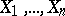is a random sample of sizefrom a population with unknown distribution functionon the real line; i.e. the's are assumed to be independent and identically distributed random variables with common distribution function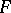(cf. also Random variable). Let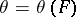denote a real-valued parameter to be estimated. Let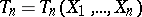denote an estimate of, based on the data(cf. also Statistical estimation; Statistical estimator). The object of interest is the probability distributionof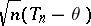; i.e.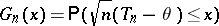for all real, the exact distribution function of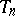, properly normalized. The scaling factor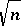is a classical one, while the centring ofis by the parameter. Here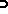denotes "probability" corresponding to.

Efron's non-parametric bootstrap estimator ofis now given by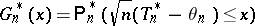for all real. Here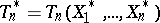, wheredenotes an artificial random sample (the bootstrap sample) from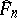, the empirical distribution function of the original observations, and. Note thatis the random distribution (a step function) which puts probability massat each of the's (), sometimes referred to as the resampling distribution;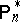denotes "probability" corresponding to, conditionally given, i.e. given the observations. Obviously, given the observed valuesin the sample,is completely known and (at least in principle)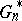is also completely known. One may viewas the empirical counterpart in the "bootstrap world" toin the "real world" . In practice, exact computation ofis usually impossible (for a sampleofdistinct numbers there are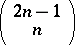distinct bootstrap (re)samples), butcan be approximated by means of Monte-Carlo simulation (cf. also Monte-Carlo method). Efficient bootstrap simulation is discussed e.g. in [a2], [a10].

When does Efron's bootstrap work? The consistency of the bootstrap approximation, viewed as an estimate of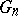, i.e. one requiresto hold, in-probability, is generally viewed as an absolute prerequisite for Efron's bootstrap to work in the problem at hand. Of course, bootstrap consistency is only a first-order asymptotic result and the error committed whenis estimated bymay still be quite large in finite samples. Second-order asymptotics (Edgeworth expansions; cf. also Edgeworth series) enables one to investigate the speed at which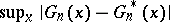approaches zero, and also to identify cases where the rate of convergence is faster than, the classical Berry–Esseen-type rate for the normal approximation. An example in which the bootstrap possesses the beneficial property of being more accurate than the traditional normal approximation is the Student-statistic and more generally Studentized statistics. For this reason the use of bootstrapped Studentized statistics for setting confidence intervals is strongly advocated in a number of important problems. A general reference is [a7].

When does the bootstrap fail? It has been proved [a1] that in the case of the mean, Efron's bootstrap fails whenis the domain of attraction of an-stable law with(cf. also Attraction domain of a stable distribution). However, by resampling from, with (smaller) resample size, satisfying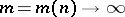and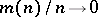, it can be shown that the (modified) bootstrap works. More generally, in recent years the importance of a proper choice of the resampling distribution has become clear, see e.g. [a5], [a9], [a10].

The bootstrap can be an effective tool in many problems of statistical inference; e.g. the construction of a confidence band in non-parametric regression, testing for the number of modes of a density, or the calibration of confidence bounds, see e.g. [a2], [a4], [a8]. Resampling methods for dependent data, such as the "block bootstrap" , is another important topic of recent research, see e.g. [a2], [a6].

How to Cite This Entry:
Bootstrap method. Encyclopedia of Mathematics. URL: http://encyclopediaofmath.org/index.php?title=Bootstrap_method&oldid=11753
This article was adapted from an original article by Roelof Helmers (originator), which appeared in Encyclopedia of Mathematics - ISBN 1402006098. See original article